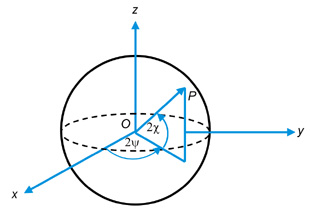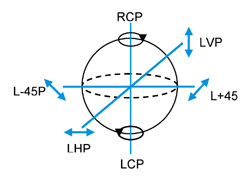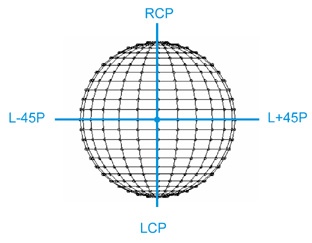# The Poincaré Sphere

Excerpt from Field Guide to Polarization

By itself, the polarization ellipse is an excellent way to visualize polarized light. However, except for the degenerate polarization states, it is practically impossible to determine the orientation and ellipticity angles viewing the polarization ellipse. Furthermore, the calculations required to determine the new angles of a polarized beam that propagates through one or more polarizing elements are difficult and tedious.

In order to overcome these difficulties Poincaré (1892) suggested using a sphere now known as the Poincaré sphere to represent polarized light. The following figure shows the Poincaré sphere and its spherical and Cartesian coordinates.Here x, y, and z are Cartesian coordinate axes, ψ and χ are the spherical orientation and ellipticity angles (of the polarization ellipse), and P is a point on the surface of the sphere. Note that on the sphere the angles are expressedas 2ψ and 2χ. For a unit sphere the Cartesian coordinates are related to the spherical coordinates by the equation.

x = cos(2χ) cos(2ψ), 0≤ ψ < π,
y = cos(2χ) sin(2ψ) , −π/4< χ ≤ π/4
z = sin(2χ),

where x2+y2+z2=1 for a sphere of unit radius.

## Degenerate States on the Poincaré Sphere

From the previous equations any polarization state can be represented by the coordinate pair (2ψ,2χ). The degenerate polarization states on the Poincaré sphere are for LHP(0°,0°), for L+45P(+90°,0°), forLVP(180°,0°), for L-45P(270°,0°), for RCP(0°,+90°), and for LCP(0°,-90°). The degenerate states on the x, y, and z axes are shown below.

All linear polarization states lie on the equator and right and left circular polarization states are at the north and south poles, respectively. Elliptically polarized states are represented everywhere else on the surface of the sphere.

The following figure shows polarization states plotted at every intersection of the 7.5° latitude and 15° longitude lines.Citation:

E. Collett, Field Guide to Polarization, SPIE Press, Bellingham, WA (2005).